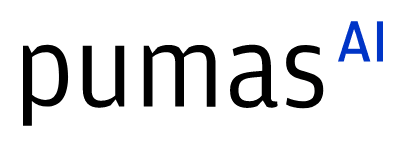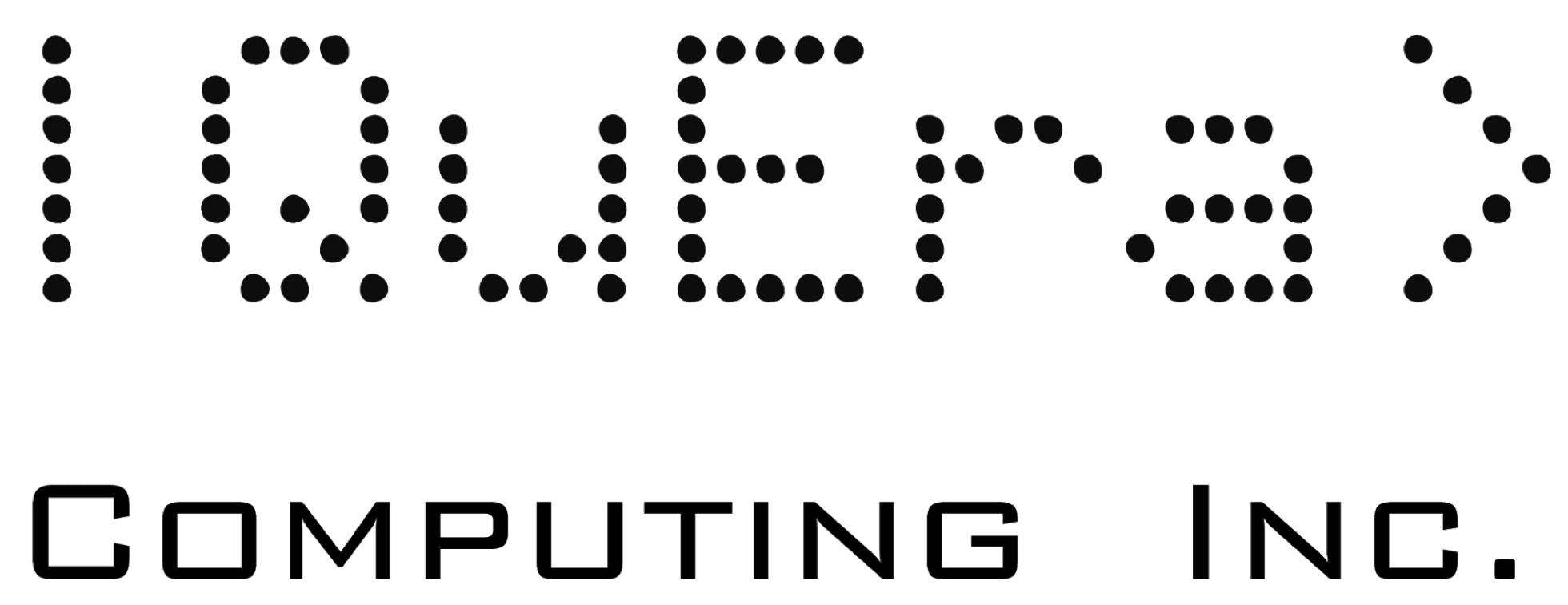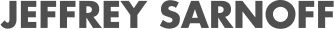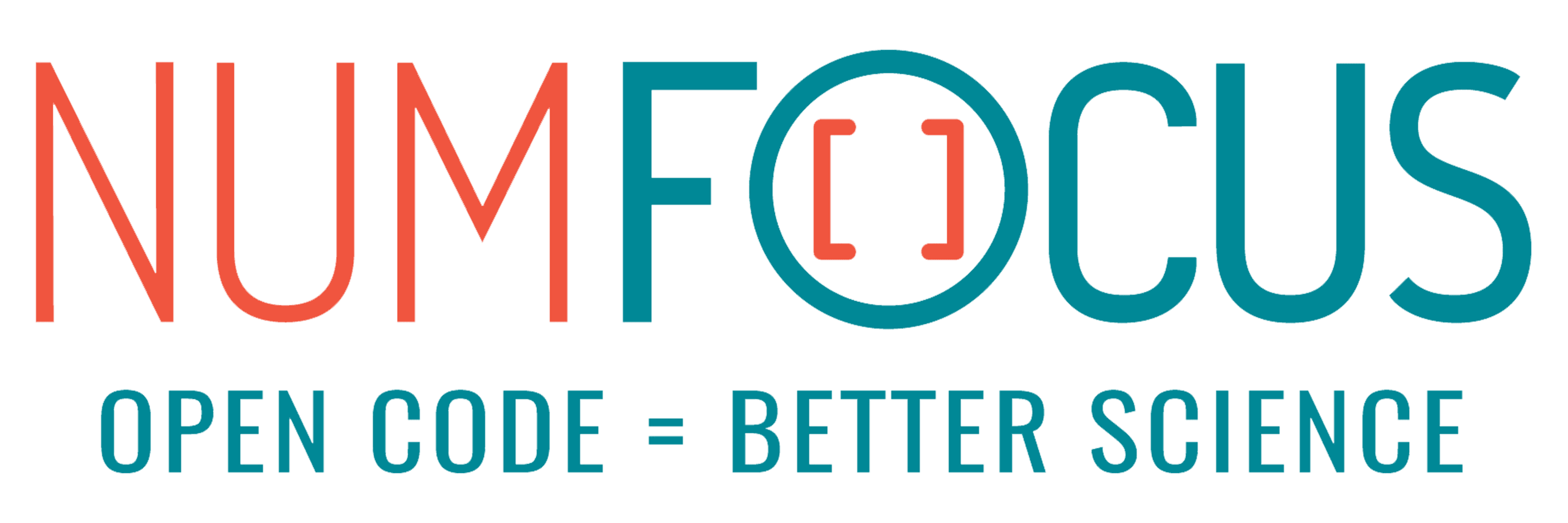# Temporal Network analysis with Julia SciML and DotProductGraphs

07/28/2023, 4:20 PM4:30 PM UTC
32-D463 (Star)

#### Abstract:

Many complex networks present a temporal nature, e.g. Social Networks, and their modelling is still an challenge. In this talk we’ll show how, in our research group, we use the Julia's SciML ecosystem to understand and predict the temporal evolution of networks. In particular, we'll present a new package DotProductGraphs.jl, that implements tools from the statistical theory of graph embedding (RDPG) to model temporal networks as dynamical systems.

#### Description:

Introduction

Complex networks can change over time as vertices and edges get added or removed. Modelling the temporal evolution of networks and predicting their structure is an open challenge across a wide variety of disciplines: from the study of ecological networks as food-webs, to predictions about the structure of economical networks; from the analysis of Social Networks, to the modelling of how our brain develops and adapts during our lives.

In their usual representation, networks are binary (an edge is either observed or not), sparse (each vertex is linked to a very small subset of the network), large (up to billions of nodes), and changes are discrete rewiring events. These properties make them hard to handle with classic machine learning techniques and have barred the use of some mathematical modelling frameworks such as differential equations. In this talk, we show how we used Julia, and in particular the Scientific Machine learning (SciML) framework, to model the temporal evolution of complex networks as continuous, multivariate, dynamical systems from observational data. We took an approach cutting across different mathematical disciplines (machine learning, differential equations, and graph theory): this was possible largely thanks to the integration of packages like Graph.jl (and the integrated MetaGraph.jl package) with the SciML ecosystem, e.g., DiffEqFlux.jl, via graph embeddings in metric spaces.

For this latter task, we will present a novel Julia package called DotProductGraphs.jl to easily and efficiently compute graph embedding, building on interpreting networks as Random Dot Product Graphs (RDPG).

Methodology

1. To translate the discrete, high-dimensional, dynamical system into a continuous, low-dimensional one, we rely on a network embedding technique. A network embedding maps the vertices of a network to points in a (low-dimensional) metric space. We adopt the well-studied Random Dot-Product Graphs statistical model: the mapping is provided by a truncated Singular Value Decomposition of the network’s adjacency matrix; to reconstruct the network we use the fact that the probability of interaction between two vertices is given by the dot product of the points they map to. The decomposition of the adjacency matrices and their alignment is a computationally intensive step, and we tackle it thanks to the fast matrix algorithms available for Julia implemented in our novel DotProductGraphs.jl package.

2. In the embedding framework, a discrete change in the network is modelled as the effect of a continuous displacement of the points in the metric space. Our goal then, is that of discovering from the data (the network observed at various points in time) an adequate dynamical system capturing the laws governing the temporal evolution of the complex network. This is possible thanks to a pipeline that combines Neural or Universal ODEs and the identification of nonlinear dynamical systems, e.g. SiNDY.

In general, each node may influence the temporal evolution of every over node in the network, and, if we are working in a space with dimension d, and N nodes, this translates to a dynamical system with NNd variables. As networks may often have thousands or millions of nodes, that number can be huge. In our talk we are going to discuss various strategies we adopted to tame the complexity of the dynamical system.

Future Development

We are now working on three fronts:

• we are scaling up the framework, so to model larger networks (for example to model social networks, where being able to predict what people might link with others in the future could be a tool to fight the growing problem of misinformation and disinformation);
• we are progressively moving from Neural Differential Equations to Universal Differential Equations, both to capture any preexisting knowledge of the network dynamical system, and to help with the training complexity;
• we are exploring data augmentation techniques to interpolate between the estimated embeddings, other embeddings, and other neural network architectures.

These three development directions constitute interesting challenges for the Julia practitioner interested in extending Julia modeling abilities. We will discuss them in the talk and suggest how everyone can contribute

All the code will be made available in a dedicated git repository and we are preparing a detailed publication to illustrate our approach.